Courses

# Simple Harmonic Motion NAT Level - 1

## 10 Questions MCQ Test Topic wise Tests for IIT JAM Physics | Simple Harmonic Motion NAT Level - 1

Description
This mock test of Simple Harmonic Motion NAT Level - 1 for IIT JAM helps you for every IIT JAM entrance exam. This contains 10 Multiple Choice Questions for IIT JAM Simple Harmonic Motion NAT Level - 1 (mcq) to study with solutions a complete question bank. The solved questions answers in this Simple Harmonic Motion NAT Level - 1 quiz give you a good mix of easy questions and tough questions. IIT JAM students definitely take this Simple Harmonic Motion NAT Level - 1 exercise for a better result in the exam. You can find other Simple Harmonic Motion NAT Level - 1 extra questions, long questions & short questions for IIT JAM on EduRev as well by searching above.
*Answer can only contain numeric values
QUESTION: 1

### A spring has a certain mass suspended from it and its period for vertical oscillation is T1. The spring is now cut into two equal halves and the same mass is suspended from one of the half. The period of vertical oscillation is now T2. The ratio of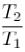is :

Solution: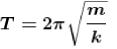When spring is cut in equal part, then force constant is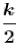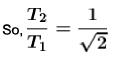*Answer can only contain numeric values
QUESTION: 2

### For a simple harmonic vibrator of frequency n, the frequency of kinetic energy changing completely to potential energy is αn. Find the value of α.

Solution:

2n

*Answer can only contain numeric values
QUESTION: 3

### The total energy of the body executing S.H.M is E. Then the kinetic energy when the displacement is half of the amplitude is αE Find the value of α.

Solution:

Kinetic energy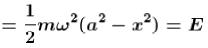Kinetic energy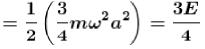*Answer can only contain numeric values
QUESTION: 4

The amplitude of a damped harmonic oscillator become halved in 1 minute. After three minutes the amplitude will become 1/x of initial amplitude where x is :

Solution:

The variation in amplitude of a damped harmonic oscillation with time is given by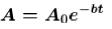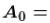initial amplitude,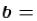damped factor
It is given that after 1 minutes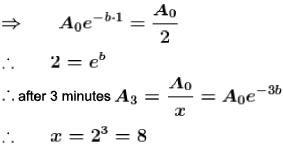*Answer can only contain numeric values
QUESTION: 5

One body of mass m is suspended from three springs as shown in figure each spring has spring constant k. If mass m is displaced slightly then time period of oscillation is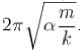Find the value of  α.Solution:

Using series and parallel combination of spring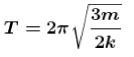*Answer can only contain numeric values
QUESTION: 6

A particle is executing SHM with an amplitude 4 cm. The displacement (in cm) at which its energy it half kinetic and half potential is :

Solution: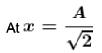Potential energy = Kinetic energy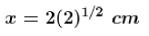*Answer can only contain numeric values
QUESTION: 7

A particle executing S.H.M of amplitude 4 cm and T = 4 sec. The time taken (in sec) by it to move from positive extreme position to half the amplitude is :

Solution: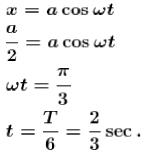*Answer can only contain numeric values
QUESTION: 8

The potential energy of a particle executing S.H.M. is 2.5 J when its displacement is half of amplitude. The total energy (in Joule) of the particle is :

Solution:

Total energy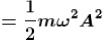Potential energy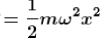So total energy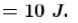*Answer can only contain numeric values
QUESTION: 9

If the time period of oscillation of mass M suspended from a spring is one second, then the time period (in sec) of 4M will be :

Solution: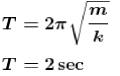*Answer can only contain numeric values
QUESTION: 10

A linear harmonic oscillation of force constant 2 x 106 Nlm and amplitude 0.01 m has a total mechanical energy of 160 joules. Its maximum K.E. (in Joule) will be.

Solution:

Maximum kinetic energy is 100 J
Maximum kinetic energy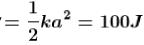Track your progress, build streaks, highlight & save important lessons and more!

### Similar Content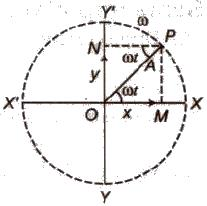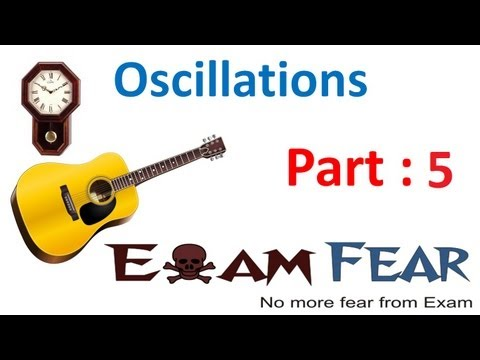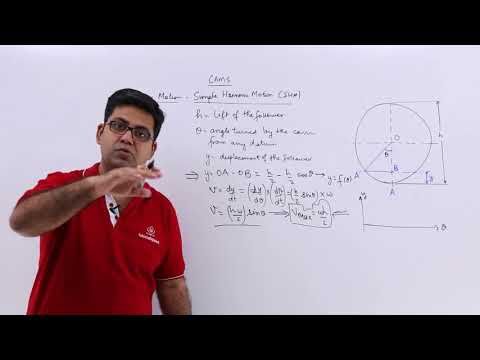### Related tests